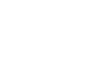What are some important questions for BSc 1st year 2nd semester questions? - letsdiskussdeepak pandit

@letsuser | Posted on | Education

What are some important questions for BSc 1st year 2nd semester questions?

0
0

Content writer and teacher also | Posted on

In BSc exams, different types of questions are asked. If we discuss chemistry, you have to prepare conceptual questions and read the book thoroughly. Generally, theory-based questions are more important in BSc exams. Practice previous 10 years paper will help you a lot.
Here are some important questions from each part of Chemistry (Inorganic Chemistry, Organic Chemistry and Physical Chemistry).
Inorganic Chemistry:
• MOT (Molecular Orbital Theory), and how does MOT id different from VBT ( Valence Bond Theory)?
• Write a short note on bond Energy.
• Define Fajan's Rule with its application?
• Explain Lattice energy
• VSEPR Theory
• S-block elements, p-block elements
• Froth Flotation Process
• A short note on Wave-Particle Duality of matter
• What do you mean by Wave Mechanical concept of Atomic Structure?
• Heisenberg’s Uncertainty Principle?
• Define Probability Density?
• The shape of Orbitals? Discuss the shapes of s, p, d- orbitals?
• Describe Schrodinger’s wave equation for Hydrogen atom?
• Write a note on Pauli’s Exclusion Law? And also write its application?
• What is Hybridization? It's Rules? And it's typed?
• Differentiate between Polar and Nonpolar Covalent Bonds?
• Define Electrovalent, organic bond, electrovalency?
• Vander Waal Forces? Its nature and sources?
• The position of Alkali Earth Metals in the Periodic table?
• What are organometallic compounds of Li?
• The position of Inert Gases in the Periodic Table?
• How can we separate Hydrogen and Nitrogen from the air?
• Explain Inert Pair Effect?
• Structure of Boric acid?
• Preparation method of carbon dioxide and its Uses?
• Haber’s Method of ammonia, its uses and properties?
• Define Hypo with its uses and properties?
• Oxyacids of Iodine?
• Physical Chemistry:
• Kinetic Theory of Ideal Gas and its Postulates?
• Derive Various Gas Laws from Kinetic Gas equation?
• Differentiate between real gas and ideal gas?
• Define Critical Phenomena?
• Maxwell Distribution Law of molecular Speed?
• Describe the Liquefaction of Gases?
• Structural Difference among solids, liquids and gases?
• Structure of liquids, explain the Theories of Liquid state?
• What do you mean by Intermolecular Forces?
• Define Liquid Crystals? Why are they called liquid crystals?
• What are amorphous solids and how they are different from crystalline?
• What is Crystallography? Explain the laws of crystallography?
• Bragg’s Equation for diffraction of X-Rays by Crystals and discuss its application?
• Differentiate between Lyophilic and Lyophobic?
• Explain the preparation methods of Colloidal Sol? And it's properties? Application?
• Emulsion and its preparation methods and types?
• The velocity of Reaction? What are the factors which affect the rate of Reaction?
• Independence of reaction rate on concentration?
• Differentiate between Molecularity and order of reaction?
• Effect of temperature on the rate of reaction?
• What is the energy of activation?
• Describe Photochemical Reaction?
• What is the fast reaction? And how their mechanism is determined?
• The assumption of Simple collision theory of reaction rates?
• Explain Catalysts and its types and uses?
• Catalytic reaction?
• Enzyme catalyst of biochemical catalysts.
• Chloroform?
• Organic Chemistry:
• Nature of covalent bond?
• Difference between sigma and pi bond and which is stronger and why?
• Tetravalency of carbon?
• Resonance and resonance energy?
• Inductive effect?
• Inclusion compounds, and discuss inclusion compound of urea?
• Difference between Hemolytic Fission and heterolytic fission?
• Free Radicals and its stability?
• Arenas? Its structure and reaction?
• Wurtz fitting reaction with mechanism?
• Geometrical Isomerism and its reasons?
• Conformation? Draw all the conformation structures?
• What is Glycol?
• Methods of formation of alcohol dehydration?
• What are toluene and its preparation methods?
• Gatterman reaction?
• Aldol reaction?
• Flying Wedge Formula?
• Olefins?
• Why do alkenes give addition reactions?
• Benzene and its homologues?
• Nucleophilic substitution reaction?
• Styrene? (Uses and properties)?
• Polynuclear hydrocarbons?
• Above are some important topics for chemistry B.Sc. 2nd Semester.

0
0